• 2021-06-14 22:11:32

当前pyecharts的版本为1.9.0。

## 概述

pyecharts/charts/composite_charts/包中的四个模块分别定义了组合图表类，其中pyecharts/charts/composite_charts/page.py模块只定义了顺序类Page

Page类继承自图表基类CompositeMixin，作用为在一个容器中顺序显示多个图表。输出的HTML结构如下：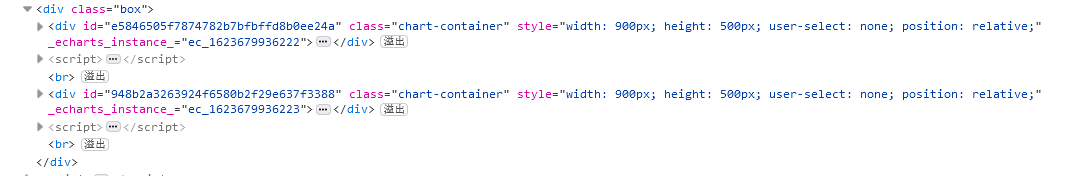Page类的签名为class Page(page_title: str = "Awesome-pyecharts", js_host: str = "", interval: int = 1, layout: Union[PageLayoutOpts, dict] = PageLayoutOpts())

Page类的构造方法参数如下：

• js_host：JavaScript库的URL。字符串，默认值为""
• page_title：HTML页面标题。字符串，默认值为"Awesome-pyecharts"
• interval：图表间隔（<br>标签个数）。整数，默认值为1
• layout：布局配置。PageLayoutOpts对象或字典，默认值为PageLayoutOpts()Page类支持三种布局模式：默认布局、SimplePageLayout布局、DraggablePageLayout布局。

Page类的类变量如下：

• SimplePageLayout：简单布局模式。值为 PageLayoutOpts( justify_content="center", display="flex", flex_wrap="wrap")
• DraggablePageLayout ：可拖拽模式。值为PageLayoutOpts()

Page类的属性如下：

• js_host：JavaScript库的URL。字符串，默认值为全局变量CurrentConfig.ONLINE_HOST。属性值为构造方法参数js_host与全局变量CurrentConfig.ONLINE_HOST进行或操作的结果。
• page_title：HTML页面标题。字符串，默认值为全局变量CurrentConfig.PAGE_TITLE
• page_interval：每个图表之间的间隔。值为构造方法参数interval值。整数。即在HTML文档中插入的<br/>标签个数。
• layout ：布局配置字符串。
• download_button：是否显示下载按钮。布尔值。默认值为False
• js_functions：自定义JavaScript语句。类型为OrderedSet对象。默认值为OrderedSet()
• js_dependencies：定义JavaScript依赖库。类型为OrderedSet对象。默认值为OrderedSet("echarts")
• _charts：容器中图表实例列表。类型为列表。默认值为[]

Page类的方法如下：

• add(*chart)：将图表类实例添加页面中。方法可接收任意个图表类实例。
• render：调用renderengine模块中的render函数渲染HTML文档。默认渲染模板为"simple_page.html"
• render_embed：调用renderengine模块中的render_embed函数输出HTML字符串。默认渲染模板为"simple_page.html"
• render_notebook：调用renderengine模块中的render_notebook函数将输出嵌入到notebook中。默认渲染模板为 "nb_jupyter_notebook_page.html""nb_jupyter_lab_page.html"

## 简易选项卡Page案例

from pyecharts.charts import Line, Page

a = ["衬衫", "羊毛衫", "雪纺衫", "裤子", "高跟鞋", "袜子"]
b1 = [5, 20, 36, 10, 75, 90]
b2 = [7, 25, 31, 14, 65, 100]

line1 = Line()

line2 = Line()

page = Page()
page.render()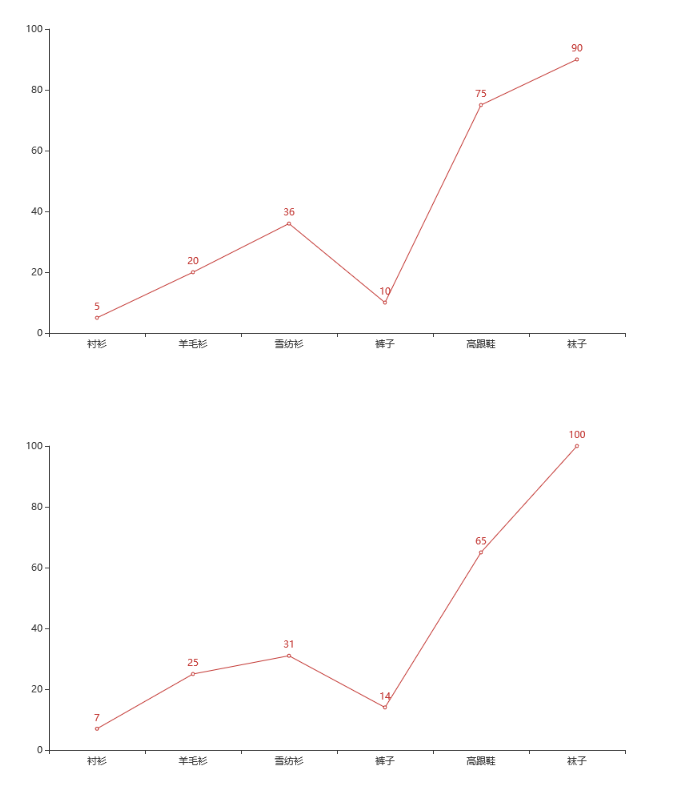pyecharts charts page
更多相关内容
• ## Seaborn多图组合

千次阅读 2019-03-02 13:05:01
数据分析中常用做图的方式实现相关性分析，即X轴设置为变量A，Y轴设置为变量B，做散点图，由于散点图中点的叠加显示，往往还需要关注每个变量自身的分布情况，jointplot把描述变量的分布图和变量相关的散点图组合...

1. jointplot 两变量图

数据分析中常用做图的方式实现相关性分析，即X轴设置为变量A，Y轴设置为变量B，做散点图，由于散点图中点的叠加显示，往往还需要关注每个变量自身的分布情况，jointplot把描述变量的分布图和变量相关的散点图组合在一起，是相关性分析最常用的工具，图片上还能展示回归曲线，以及相关系数。

import statsmodels.api as sm
import seaborn as sns
sns.set(style="darkgrid")
g = sns.jointplot('AVGEXP', 'AGE', data=data, kind="reg",
xlim=(0, 1000), ylim=(0, 50), color="m")本例中使用statsmodels库的ccard数据，分析了其中两个数值类型变量的相关性，使用xlim和ylim设置了图片显示范围，忽略了离群点，kind参数可设置做图方式：scatter散点图，kde密度图，hex六边形图等，本例中选择reg画出了线性回归图。

2. pairplot多变量图

如果用对N个变量的相关性做散点图，用maplotlib需要做NxN个图，用pairplot函数调用一次即可实现，其对角线上是直方图，其余都是两两变量的散点图，不仅简单，还能组合在一起作对比。

data = sm.datasets.ccard.load_pandas().data
sns.pairplot(data, vars=['AGE','INCOME', 'INCOMESQ','OWNRENT'])图中可以看到，数据类型INCOME与INCOMESQ呈强相关，AGE与INCOME也有一定相关趋势，对角线上的图对应的是每个因素与其自身的对比，图中以直方图显示了该变量的分布。

3. factorplot两变量关系图

factorplot用于绘制两维变量的关系图，用kind可指定其作图类型，包括：point, bar, count, box, violin, strip等。

data = sm.datasets.fair.load_pandas().data
sns.factorplot(x='occupation', y='affairs', hue='religious', data=data)4. FacetGrid结构化绘图网格

FacetGrid可以选择任意的做图方式，以及自定义的做图函数，通常包含两部分，FacetGrid部分指定了数据集，行，列，map部分指定做图方式，以及相应参数。

g = sns.FacetGrid(tips, col = 'time', row = 'smoker') # 按行和列的分类做N个图
g.map(plt.hist, 'total_bill', bins = 10) # 指定做图方式可以看到无论是连续图，还是分类图，无论是用FacetGrid还是barplot都是将多个特征放在同一张图片上展示，其差别一方面在于观察角度不同，另一方面也取决于数据自身的类型。

展开全文• par(mfrow=c(2,2))，可以理解将绘图区域分割为2x2的矩阵区域，另可参照《R语言实战》3.5图形的组合 ##################par(mfrow=c(2,2))#################### attach(iris) opar <- par(no.readonly = TRUE) ...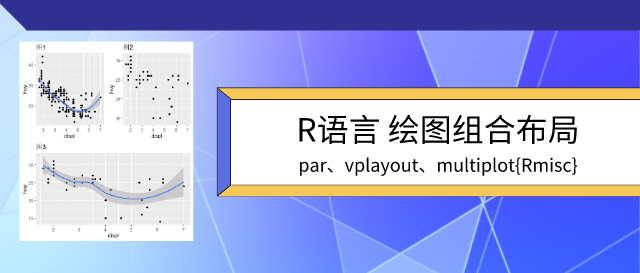01

图形组合布局

par(mfrow=c(2,2))

par(mfrow=c(2,2))，可以理解将绘图区域分割为2x2的矩阵区域，另可参照《R语言实战》3.5图形的组合

##################par(mfrow=c(2,2))####################

attach(iris)

# data(iris)

# Sepal.Length Sepal.Width Petal.Length Petal.Width Species

# 1          5.1         3.5          1.4         0.2  setosa

# 2          4.9         3.0          1.4         0.2  setosa

# 3          4.7         3.2          1.3         0.2  setosa

# 4          4.6         3.1          1.5         0.2  setosa

# 5          5.0         3.6          1.4         0.2  setosa

# 6          5.4         3.9          1.7         0.4  setosa

​par(mfrow=c(2,2)) # 设置2x2的布局

plot(Sepal.Length,Sepal.Width, main = "第一张图")

boxplot(Sepal.Length, horizontal = TRUE, main = "第二张图")

boxplot(Sepal.Width, main = "第三张图")

hist(Petal.Length, main = "第四张图")​

par(opar)

detach(iris)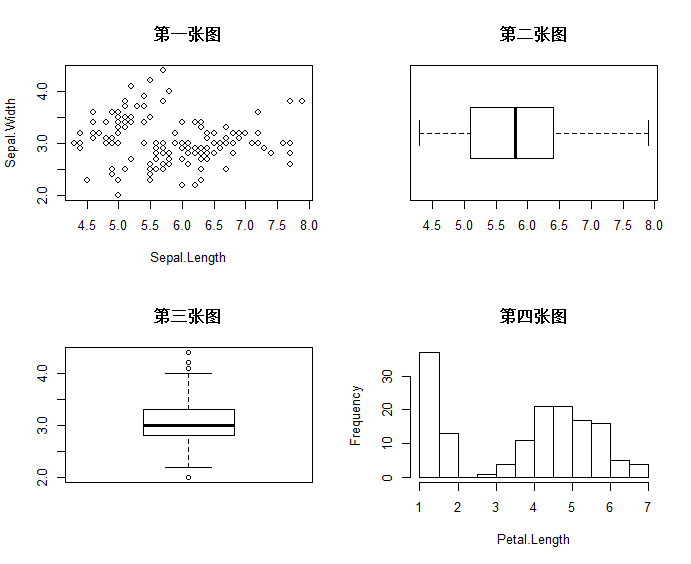02

图形组合布局

par(fig=c(x1, x2, y1, y2), new = TRUE)

par(fig=c(x1, x2, y1, y2), new = TRUE)，取x1,x2,y1,y2四条线圈住的位置绘图图形，另可参照《R语言实战》3.5图形的组合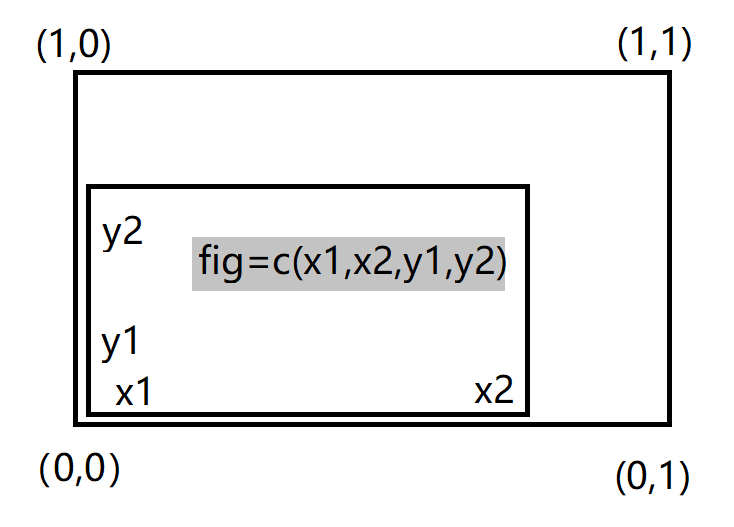par(fig=c(0,0.8,0,0.8)) # 设置散点图的布局参数

plot(iris$Sepal.Length,iris$Sepal.Width) #绘制散点图​

par(fig=c(0,0.8,0.65,1),new = TRUE) #设置上方箱型图的布局参数

boxplot(iris$Sepal.Length, horizontal = TRUE, axes=FALSE) # 绘制上方箱型图​ par(fig=c(0.65,1,0,0.8),new = TRUE) #设置右侧箱型图的布局参数 boxplot(iris$Sepal.Width, axes=FALSE) # 绘制右侧箱型图​

mtext("par(fig=c(x1, x2, y1, y2), new = TRUE)",side = 3, outer=TRUE, line=-3)

par(opar)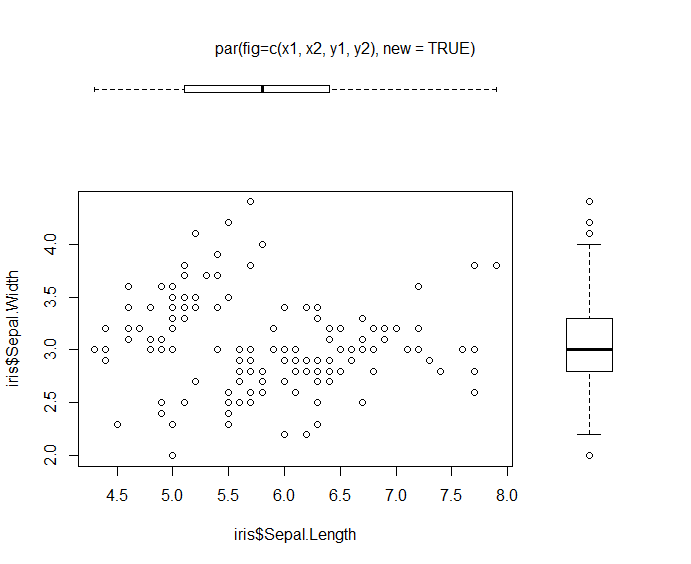03

图形组合布局

grid.layout & vplayout

library(ggplot2)

​#绘制基本ggplot图

base <- ggplot(mpg, aes(displ, hwy)) + geom_point()

p1 <- base + geom_smooth() + labs(title="图1") #如图1

​#用%+%调整映射关系中的数据

base <- ggplot(mpg, aes(displ, hwy)) + geom_point()

p2 <- base %+% subset(mpg, fl == "p") + labs(title="图2") #图2​#第二种调整数据的方法list

p3 <- base + list(subset(mpg, fl == "p"), geom_smooth(), labs(title="图3")) #图3​

###########一页多图########

library(grid)

grid.newpage()  ##新建页面

pushViewport(viewport(layout = grid.layout(2,2))) #将页面分成2*2矩阵

vplayout <- function(x,y){ viewport(layout.pos.row = x, layout.pos.col = y)}​

print(p1, vp = vplayout(1,1))   #（1,1)的位置画图1

print(p2, vp = vplayout(1,2))   #(1,2)的位置画图2

print(p3, vp = vplayout(2,1:2))  #（2,:)的位置画图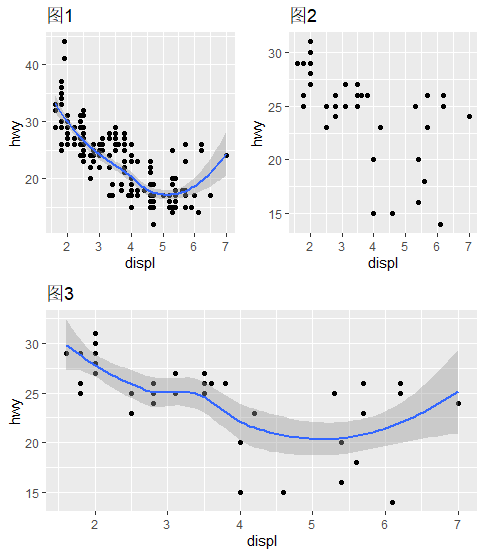04

图形组合布局

plot_grid {cowplot}

install.packages("cowplot") #安装cowplot包

library(cowplot) # 加载

?plot_grid  #帮助函数查看具体usage

示例

library(ggplot2)​

df <- data.frame(  x = 1:10, y1 = 1:10, y2 = (1:10)^2, y3 = (1:10)^3, y4 = (1:10)^4)

​p1 <- ggplot(df, aes(x, y1)) + geom_point()

p2 <- ggplot(df, aes(x, y2)) + geom_point()

p3 <- ggplot(df, aes(x, y3)) + geom_point()

p4 <- ggplot(df, aes(x, y4)) + geom_point()

gridplot_grid(p1, p2, p3, p4)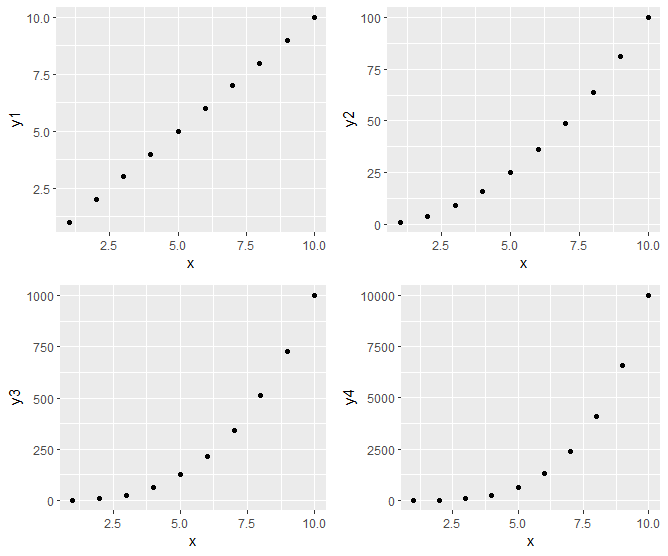05

图形组合布局

multiplot{Rmisc}

############################# multiplot{Rmisc} ##################################

library(Rmisc)

library(ggplot2)​

df <- data.frame(  x = 1:10, y1 = 1:10, y2 = (1:10)^2, y3 = (1:10)^3, y4 = (1:10)^4)​

p1 <- ggplot(df, aes(x, y1)) + geom_point()

p2 <- ggplot(df, aes(x, y2)) + geom_point()

p3 <- ggplot(df, aes(x, y3)) + geom_point()

p5 <- ggplot(mpg, aes(as.factor(year), hwy)) +  geom_boxplot() +  facet_wrap(~class, scales = "free_y")​

multiplot(p1, p2, p3, p5, cols=2)​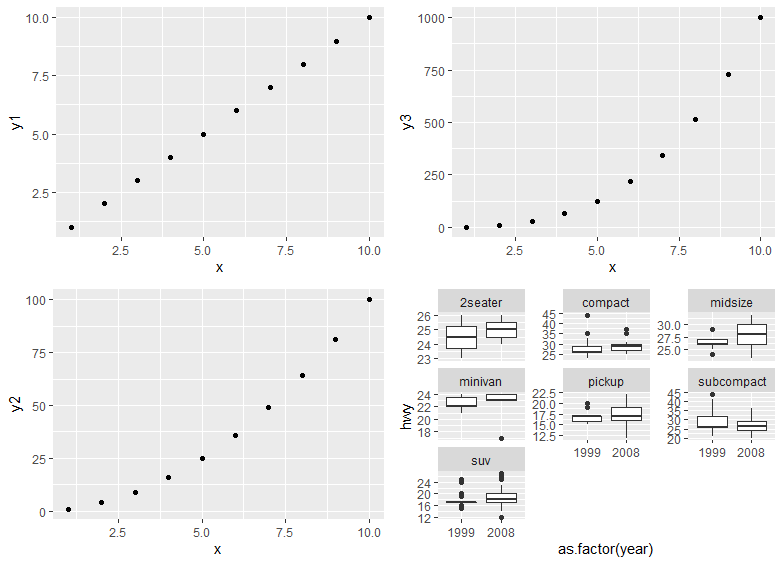获取源代码请至“数据驱动实践”公众号后台回复：一页多图

展开全文r语言
• ## ggplot2一页多图（组合图）

万次阅读 多人点赞 2019-07-16 22:57:25
suppressMessages(library(dplyr)) suppressMessages(library...#绘制第一幅p1 p1 <- diamonds %>% ggplot(aes(x = color, y = price, fill = color)) + geom_violin() + facet_grid(clarity ~ ....
suppressMessages(library(dplyr))
suppressMessages(library(ggplot2))
#绘制第一幅图p1
p1 <- diamonds %>%
ggplot(aes(x = color, y = price, fill = color)) +
geom_violin() +
facet_grid(clarity ~ .) +
theme_classic() +
scale_fill_brewer(palette = 'Set1')
p1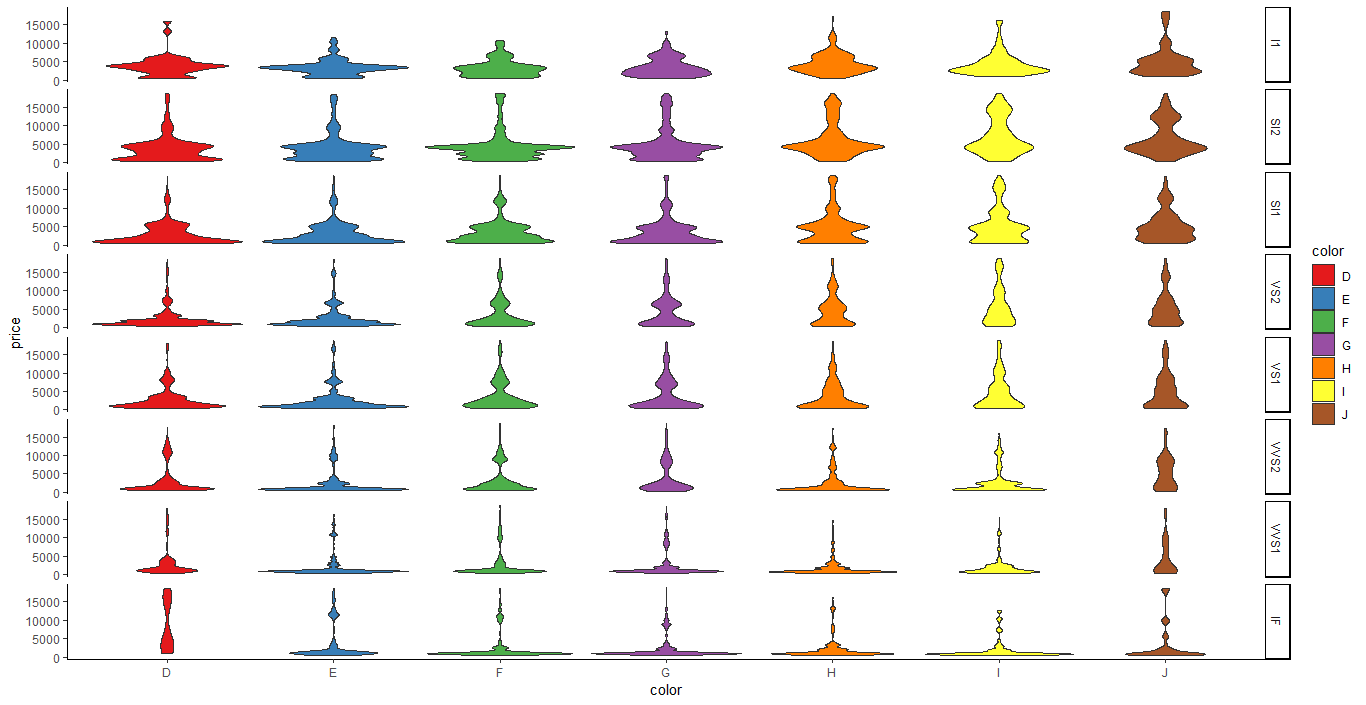#绘制第二幅图p2
p2 <- diamonds %>%
ggplot(aes(x = color, fill = clarity)) +
geom_bar(stat = 'count') +
scale_fill_brewer(palette = 'Set1') +
theme_bw()
p2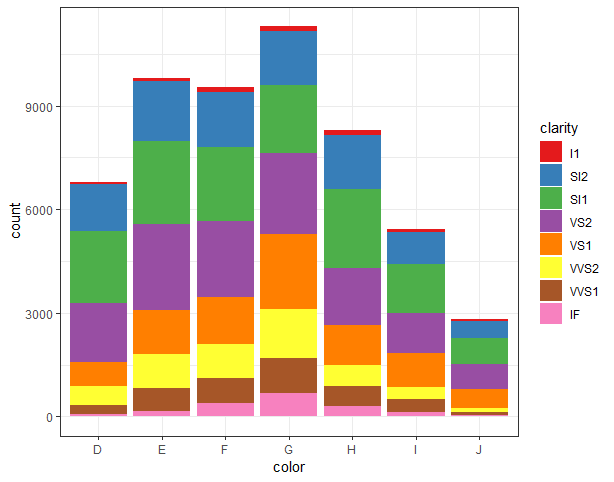#绘制第三幅图p3
p3 <- diamonds %>%
ggplot(aes(x = color, fill = clarity)) +
geom_bar(stat = 'count', position = 'fill') +
scale_fill_brewer(palette = 'Set3') +
theme_bw()
p3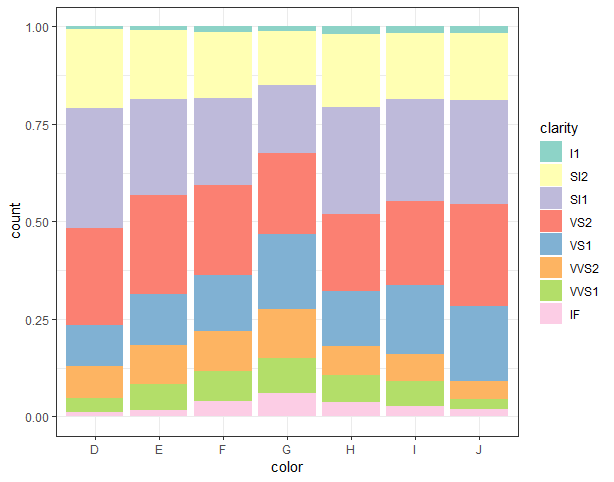#绘制第四幅图p4
p4 <- diamonds %>%
ggplot(aes(x = color, fill = clarity)) +
geom_bar(stat = 'count') +
coord_polar() +
scale_fill_brewer(palette = 'Set2') +
theme_bw()
p4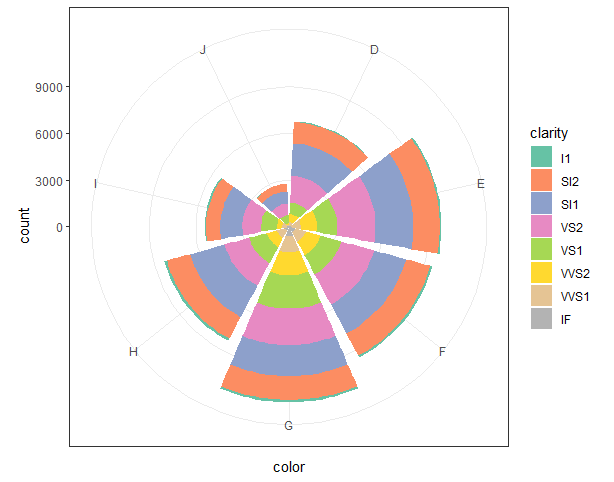组合图方法一：cowplot包plot_grid()函数

p5 <- cowplot::plot_grid(p1, p2, p3, p4, nrow = 2, labels = LETTERS[1:4])#将p1-p4四幅图组合成一幅图，按照两行两列排列，标签分别为A、B、C、D。（LETTERS[1:4] 意为提取26个大写英文字母的前四个:A、B、C、D）
p5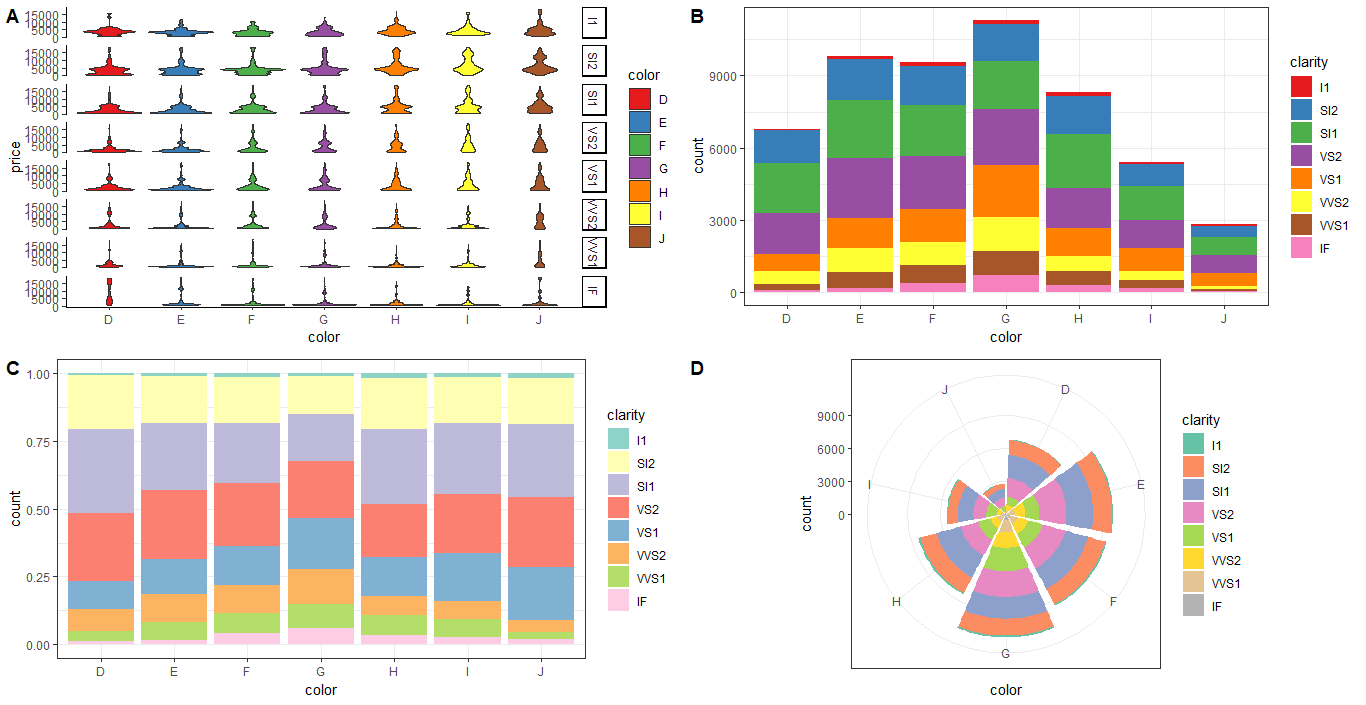组合图方法二：ggpubr包ggarrange()函数

p6 <- ggpubr::ggarrange(p1, p2, p3, p4, nrow = 2, ncol = 2, labels = c('A', 'B', 'C', 'D'), font.label = list(color = 'red'))#将p1-p4四幅图组合成一幅图，按照两行两列排列，标签分别为A、B、C、D，颜色为红色(通过font.label = list()修改)，无法通过label.color = 'red'或其他方式修改。
p6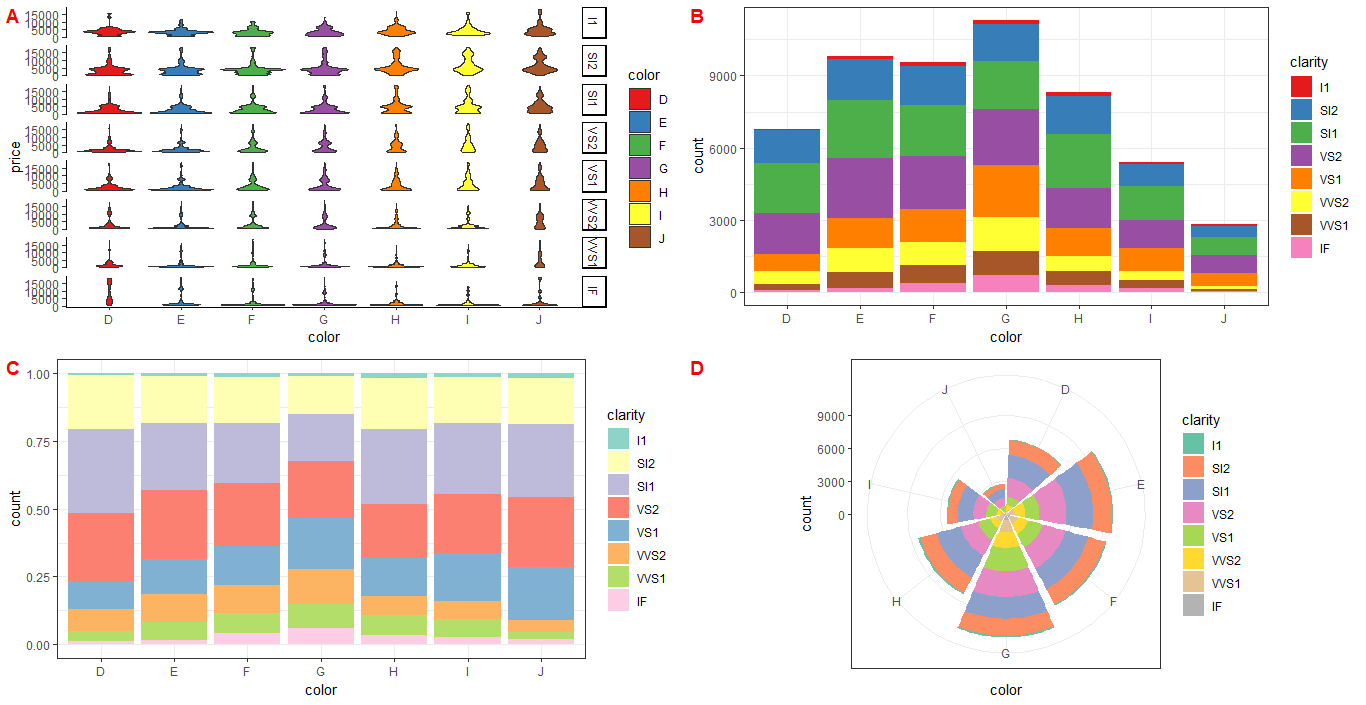展开全文R语言 ggplot2
• 在制作word文档时，有时为了使文档的内容更具有说服力，需要插入一些图片，因此会用到图片组合工具，然而有好多人却不知道如何去使用组合工具，这里小编就给大家分享在word中如何使用组合工具来组合图片。...
• ).add_yaxis( series_name='治愈数', y_axis=crued_num, yaxis_index=2, #使用的y轴的index，在单个图表实例中存在个y轴的时候有用 ) # c.添加 额外的坐标轴 bar.extend_axis( # 对y轴配置 yaxis=opts.AxisOpts( ...python
• 感谢邀请，如果要用手机把张图片拼接成一张整体的图片的话：除了以上几位答友推荐的软件之外另外其实手机上还有一款叫做“全能相机”的第三方手机相机和相片加工APP。这个APP不仅能取代手机系统原有的相机功能...
• 使用柱形图(新特性)图表如何实现堆积柱形图组合柱形图（即设置个系列堆积，其他系列为柱形图），如下图。 2. 示例 2.1 准备数据 新建内置数据集 Embedded1，如下： 2.2 插入图表 以单元格图表为例...
• 在R中使用函数par()或layout()可以容易地组合多幅图形为一幅总括图形。 你可以在par()函数中使用图形参数mfrow=c(nrows, ncols)来创建按行填充的、行数为 nrows、列数为ncols的图形矩阵。另外，可以使用nfcol=c(n...图形 r语言
• 作为EXCEL中非常重要的功能，今天给大家介绍一个柱形和折线组合图案例。如下数据源，是某一时间段6个销售员工的销售数据。现在需要在柱形上显示销售数据的平均线，并让大于平均值和小于平均值的数据显示...
• 怎样用手机将张照片拼成一张组合图？手机拼图有很方式，拼长，网格拼图。自由拼图，等。这里简单介绍几个最经典的。不用额外拼图软件相信大多数人用微信发朋友圈，却不知道微信就自带拼长功能吧。下面我们...
• 一、个波段图像组合 1.打开envi,把要组合的波段的图像都打开 打开后如上所示。 2.点击Basic Tools（基本工具）-----Layer Stacking（图层堆栈） 点击Import File,把要组合的图像都添加进去，之后点击ok。 添加...
• 文章目录Grid 组合组件 5例阅读和使用方法Demo案例及视频图表合并显示个Y轴刻度组合图折线图、柱状图组合图表柱状图、地理图组合图表折线图、散点图组合图 Grid 组合组件 5例 Python在处理各种数据时，利用图表 ...python pyecharts
• 最近研究网络中的组合优化问题时，发现已经有研究人员将神经网络用于求解此类问题，诸如寻找网络中的最大点独立集、最小点覆盖集、最大团等等，并找到了相关的源码，先马在这里，以后再抽时间介绍一下。...人工智能 强化学习 网络优化
• 实现组合绘图、个图形重合叠加、图片叠加绘图。不同的数据放到同一张图展示。
• 没有组合/联合等操作的先把功能打开 viso工具栏 文件-选项-高级-勾选 以开发人员模式运行 ...上色操作附链接吧 简单组会不需要掌握这么个图形组合时会遇到这个问题。 在开发工具里，选择-操作-visio
• 在图表中选择一个自身喜欢的组合统计类型，如下所示。 选择自身喜欢类型如下 如有不会添加数据标签的话，可以访问该文章地址：https://blog.csdn.net/qqj3066574300/article/details/107603544 然后给...
• 先把数值和百分比都做成柱形，然后在上选中百分比的系列，右键选择“更改系列图表类型”，这时百分比系列由柱形变位折线，然后再右键点击折线，选“更改数据系列格式”，在系列选项中选“次坐标轴” ...
• ## ggplot2组合绘制多个图形

万次阅读 多人点赞 2019-08-19 12:05:17
ggplot2绘制张图形为一个，进行叠加组合画布分
•uml
• 昨天教程 股评师分析报告文本情感分析预测股价 最后绘制的图片横坐标都是一样的，我想将价格与情感得分合并到一个中，这样更方便查看看趋势走势是否一致性。如下今日主要是学习如何将共享某...
• 遇到折线和柱形组合图的时候，当有数据变化，就会有相应变化，但是有的时候会出现折线和柱形重叠的时候（叠加到一起了部分数据），那么我们想要调节高低的时候应该怎么做呢？ 首先： 点击图片，右侧出现＋...excel
• 论文一般使用【AI】画，但由于我年幼无知，用PS画图，且尺寸设得很小，所以放到文章里感觉像个假，像截得。。。。 为方便，我用Visio重画，可能很多人认为它功能单一，只能画流程。其实不然visio
• 作者：李誉辉 四川大学在读研究生 前言这篇是作者总结的目前最全的R语言—图片组合和拼接，当然常言道：百密必有一疏，欢迎大家在评论区留言本篇没有总结到的用于图片组合和拼接...
• 写在正文之前 ...在SCI论文中 常常需要多图合并成一个大图 如下图所示 （我发表的第一篇论文） 以前 我是这么做的 一种是每个图都画一遍，然后在VISIO中合并调整间距另一种是用Origin2018中的四格图...
• 其实，这个图表本身并不复杂，就是两个柱状和一个曲线组合图表。但在Tableau里想要实现这样的呈现结果，还是需要一些技巧的。 本期《举个栗子》，阿达要给大家分享的Tableau技巧是：巧妙实现 双柱 和 折线...
• 前言 与前几篇类似地，笔者翻译了一篇来自海外的网络研讨会议纪要，较为简洁地为各位读者呈现了...我们在根据个因子选择策略时存在着各种各样构建组合方式，“混合”（Mixing），与“整合”（Integrating）是其...量化投资 机器学习 人工智能
• 有时候我们业务情景需要字段组合唯一性，这里举例 Navicat 设置，如所示。 Ps：但我们一般企业级开发，不推荐用物理约束死，推荐用逻辑来判断。 ...Navicat MySQL
• 七、稳步上涨 稳步上涨通常出现在股价上升趋势中，由根K线组合而成，众多阳线中间夹着较少的小阴线或十字星，股价重心不断抬高，如下所示。 稳步上涨K线组合显示上攻走势极为稳健，虽然股价在收出阳线之后紧跟...
• 图片下边加文字组合 简介 我们在开发程序的时候，很大情况会...效果 代码 wxml <view class="view_saoyisao"> <image class="image_saoyisao" src="../images/main_yewu.png"></image> <......